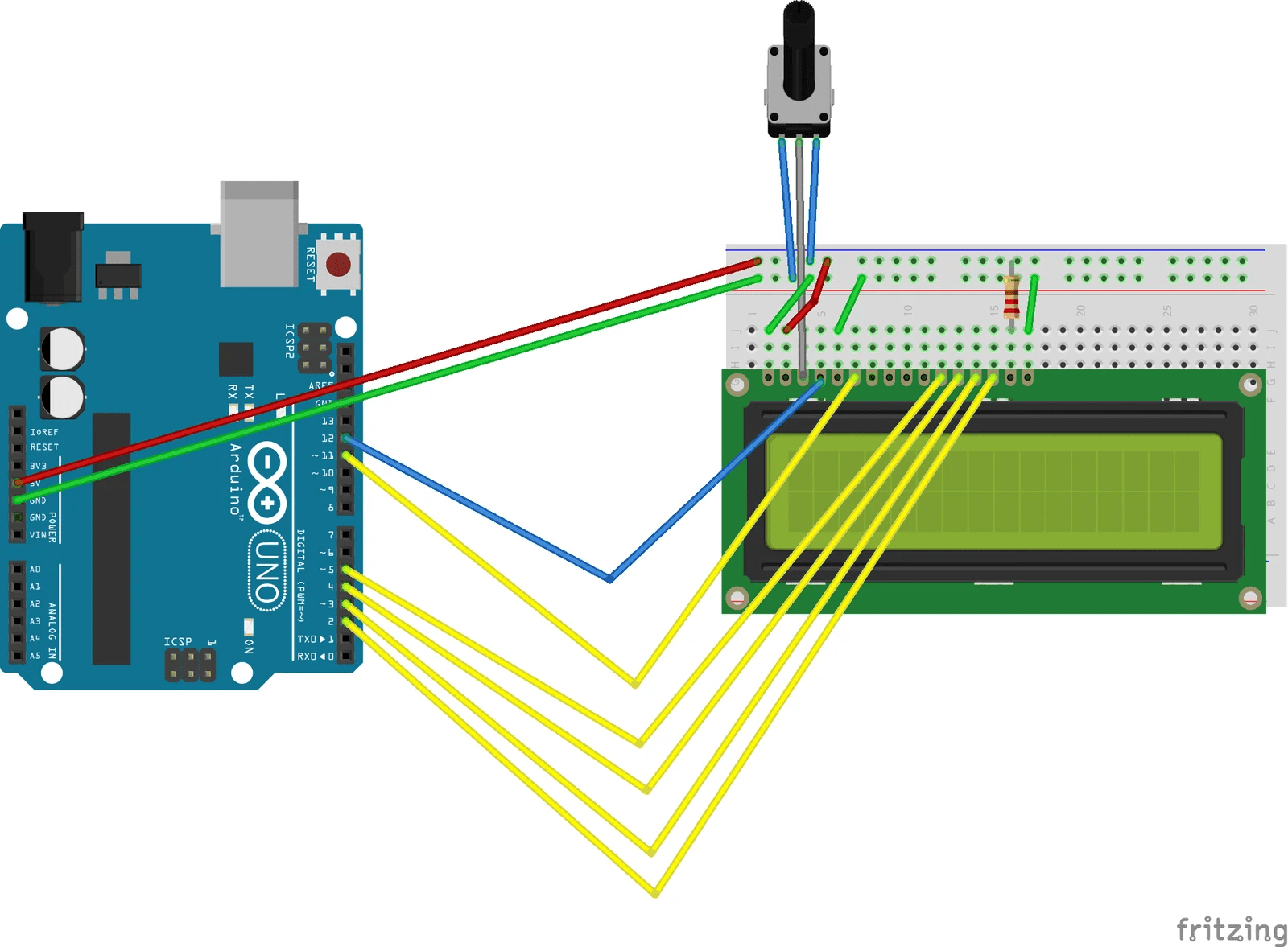# STOPWATCH USING ARDUINO AND LCD DISPLAY

In this project, we are going to make a version of a digital clock that can keep a record of hours, minutes and seconds, just like a stopwatch on your mobile phone! We are going to use an LCD to display the time.

BY ASHISH BARTWAL

LIST OF COMPONENTS REQUIRED

1.  Arduino
2.  1602 LCD Screen (16 x 2 LCD)
3.  Jumper wires
4.  Arduino program in computer
5.  220ῼ resistor
6.  10k potentiometer

CIRCUIT DIAGRAMCONNECTIONS

Make connection as shown in the circuit:

1. LCD RS pin to digital pin 12
2. LCD Enable pin to digital pin 11
3. LCD D4 pin to digital pin 5
4. LCD D5 pin to digital pin 4
5. LCD D6 pin to digital pin 3
6. LCD D7 pin to digital pin 2
7. LCD R/W pin to ground
8. 220 ῼ resistor: between LCD pin 15 and ground
9. Potentiometer wiper to LCD VO pin (pin 3) and ends to +5v and ground of Arduino kit
10. LCD pin 16 to ground

EXPLANATION OF THE CIRCUIT:

There are total 8 data pins in an LCD for providing 8-bit data input. In this project, we are going to use only 4 of them (D4-D7) as we need it to work in a 4-bit mode(Stopwatch). RS pin is the Register Select pin. It selects how and what data is to be displayed. Enable pin enables the LCD to receive data. R/W pin is used to either read or write data onto the LCD. For this project, we have set it to low (ground), so it will perform only read operations. Potentiometer acts as a variable resistor and is used to adjust the brightness of LCD.

Arduino PROGRAM:

#include <LiquidCrystal.h>                 //includes the library code of LCD in aurdino

unsigned long time;

long int seconds=0;

int minutes=0;

int hours=0;

LiquidCrystal LCD(12, 11, 5, 4, 3, 2);  //RS,EN,D4,D5,D6,D7 pin of LCD respectively

void setup()

{

Serial.begin(9600);                   //initiates serial communication

LCD.begin(16, 2);     //sets the number of columns and rows

}

void loop()

{

setClock();         //function

LCD.setCursor(0,1);     //sets the cursor to 0th column and 1st row,numbering starts from 0

{

if(hours<10)        //suppose 4

{

LCD.print(“0”);     //LCD first prints 0 and stopwatch shows 0

LCD.print(hours);       //LCD then prints 4. So value printed is 04 stopwatch shows 04

}

else

{

LCD.print(hours);

}

}

LCD.print(“:”);

{

if(minutes<10)

{

LCD.print(“0”);

LCD.print(minutes);

LCD.print(“:”);

}

else

{

LCD.print(minutes);

LCD.print(“:”);

}

}

{

if(seconds<10)

{

LCD.print(“0”);

LCD.print(seconds);

}

else

{

LCD.print(seconds);

}

}

}

void setClock()

{

seconds++;       //counts seconds from 0

delay(1000);     //a delay of 1000 milliseconds is given for each second count

if (seconds>59)

{

seconds=0;      //whenever second is greater than 59 than second is made equal to

minutes++;     // zero and a minute is counted for it

}

if (minutes>59)

{hours++;

minutes=0;

}

if(hours>23)

{

hours=0;

}

}

WORKING:

Since in one day there are 24 hours, an hour has 60 minutes and a minute has 60 seconds, Arduino first counts the number of seconds then further turn it into minutes and hours.This is the basic logic behind any stopwatch. You can further develop the code to count the number of days and weeks.

Liquid Crystal LCD(12, 11, 5, 4, 3, 2): This command sets the interfacing of LCD pin      RS,EN,D4,D5,D6,D7 to the Arduino pin 12,11,5,4,3,2 respectively.

In void setup function we initialize the serial communication and write from where data is to be displayed in LCD.

void loop()-This function checks the given condition again and again and then gives the output according to whether the condition is satisfying or not. An example stated below can explain this:

void loop()

{

if(hours<10)

{

LCD.print(“0”);

LCD.print(hours);

}

else

{

LCD.print(hours);

}

}

The above function keeps on repeating and checking whether hours is less than 10 or not.  If this condition is true then 0 is printed on LCD and then the hour. If an hour is greater than 10 then it will print the hour as it is. This function keeps on moving in a loop.

setClock– This is a function that sets a condition:  whenever the seconds is equal to 60 then Arduino count minutes and make seconds 0. Same is done in case of minutes and hours.

1.movers says:
2.Corina Etienne says:
3.Tilda Ryburn says: